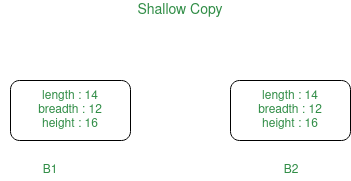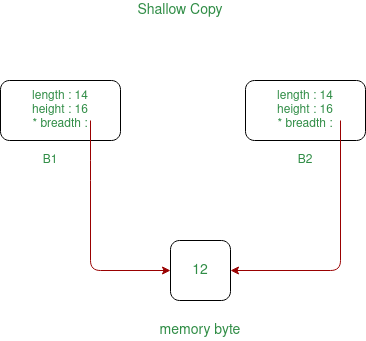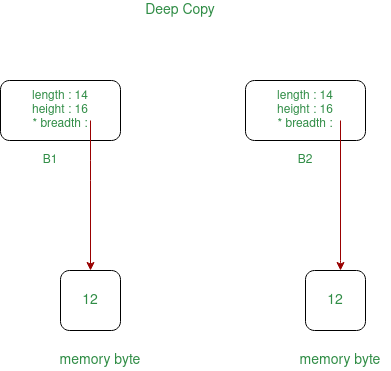# Shallow Copy and Deep Copy in C++

In general, creating a copy of an object means to create an exact replica of the object having the same literal value, data type, and resources.

// Copy Constructor
Geeks Obj1(Obj);
or
Geeks Obj1 = Obj;

// Default assignment operator
Geeks Obj2;
Obj2 = Obj1;

Depending upon the resources like dynamic memory held by the object, either we need to perform Shallow Copy or Deep Copy in order to create a replica of the object. In general, if the variables of an object have been dynamically allocated, then it is required to do a Deep Copy in order to create a copy of the object.

### Shallow Copy:

In shallow copy, an object is created by simply copying the data of all variables of the original object. This works well if none of the variables of the object are defined in the heap section of memory. If some variables are dynamically allocated memory from heap section, then the copied object variable will also reference the same memory location.
This will create ambiguity and run-time errors, dangling pointer. Since both objects will reference to the same memory location, then change made by one will reflect those change in another object as well. Since we wanted to create a replica of the object, this purpose will not be filled by Shallow copy.
Note: C++ compiler implicitly creates a copy constructor and overloads assignment operator in order to perform shallow copy at compile time.Shallow Copy of object if some variables are defined in heap memory, then:Below is the implementation of the above approach:

## C++

 `// C++ program for the above approach` `#include ` `using` `namespace` `std;`   `// Box Class` `class` `box {` `private``:` `    ``int` `length;` `    ``int` `breadth;` `    ``int` `height;`   `public``:` `    ``// Function that sets the dimensions` `    ``void` `set_dimensions(``int` `length1, ``int` `breadth1,` `                        ``int` `height1)` `    ``{` `        ``length = length1;` `        ``breadth = breadth1;` `        ``height = height1;` `    ``}`   `    ``// Function to display the dimensions` `    ``// of the Box object` `    ``void` `show_data()` `    ``{` `        ``cout << ``" Length = "` `<< length` `             ``<< ``"\n Breadth = "` `<< breadth` `             ``<< ``"\n Height = "` `<< height` `             ``<< endl;` `    ``}` `};`   `// Driver Code` `int` `main()` `{` `    ``// Object of class Box` `    ``box B1, B3;`   `    ``// Set dimensions of Box B1` `    ``B1.set_dimensions(14, 12, 16);` `    ``B1.show_data();`   `    ``// When copying the data of object` `    ``// at the time of initialization` `    ``// then copy is made through` `    ``// COPY CONSTRUCTOR` `    ``box B2 = B1;` `    ``B2.show_data();`   `    ``// When copying the data of object` `    ``// after initialization then the` `    ``// copy is done through DEFAULT` `    ``// ASSIGNMENT OPERATOR` `    ``B3 = B1;` `    ``B3.show_data();`   `    ``return` `0;` `}`

Output:

```Length = 14
Height = 16
Length = 14
Height = 16
Length = 14
Height = 16```

### Deep Copy:

In Deep copy, an object is created by copying data of all variables, and it also allocates similar memory resources with the same value to the object. In order to perform Deep copy, we need to explicitly define the copy constructor and assign dynamic memory as well, if required. Also, it is required to dynamically allocate memory to the variables in the other constructors, as well.Below is the implementation of the above approach:

## C++

 `// C++ program to implement the` `// deep copy` `#include ` `using` `namespace` `std;`   `// Box Class` `class` `box {` `private``:` `    ``int` `length;` `    ``int``* breadth;` `    ``int` `height;`   `public``:` `    ``// Constructor` `    ``box()` `    ``{` `        ``breadth = ``new` `int``;` `    ``}`   `    ``// Function to set the dimensions` `    ``// of the Box` `    ``void` `set_dimension(``int` `len, ``int` `brea,` `                       ``int` `heig)` `    ``{` `        ``length = len;` `        ``*breadth = brea;` `        ``height = heig;` `    ``}`   `    ``// Function to show the dimensions` `    ``// of the Box` `    ``void` `show_data()` `    ``{` `        ``cout << ``" Length = "` `<< length` `             ``<< ``"\n Breadth = "` `<< *breadth` `             ``<< ``"\n Height = "` `<< height` `             ``<< endl;` `    ``}`   `    ``// Parameterized Constructors for` `    ``// for implementing deep copy` `    ``box(box& sample)` `    ``{` `        ``length = sample.length;` `        ``breadth = ``new` `int``;` `        ``*breadth = *(sample.breadth);` `        ``height = sample.height;` `    ``}`   `    ``// Destructors` `    ``~box()` `    ``{` `        ``delete` `breadth;` `    ``}` `};`   `// Driver Code` `int` `main()` `{` `    ``// Object of class first` `    ``box first;`   `    ``// Set the dimensions` `    ``first.set_dimension(12, 14, 16);`   `    ``// Display the dimensions` `    ``first.show_data();`   `    ``// When the data will be copied then` `    ``// all the resources will also get` `    ``// allocated to the new object` `    ``box second = first;`   `    ``// Display the dimensions` `    ``second.show_data();`   `    ``return` `0;` `}`

Output:

```Length = 12